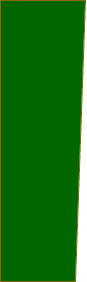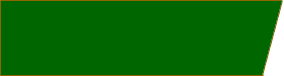Quandaries and Queries We are trying to calculate the square footage of a section of land with the following dimensions: One end is 100 feet. The other end is 105 feet.. One side is 350 feet and the other side is 372 feet. I calculated the area as 35,875 sq. feet, however, the brochure for the land says the area is 36,200 + sq. feet. Help! Diane Hi Diane, I drew two different diagrams for this problem. In the first diagram I drew the two shorter sides are parallel and when I calculated the area, I got the answer you got: 35,875 sq. ft.In the second diagram I drew the two longer sides parallel and when I calculated the area, I got 36,100 sq. ft.The area of the land would depend on not just the dimensions, but the shape as well. For example if we knew the length of one of the diagonals, or the measure of one of the angles, or if two sides are parallel, (if we knew which two), then we can find the area. Leeanne Go to Math Central